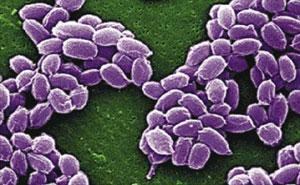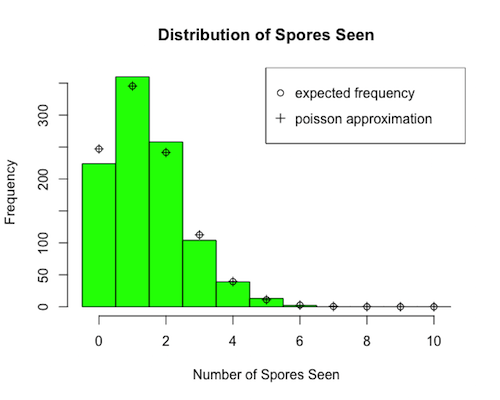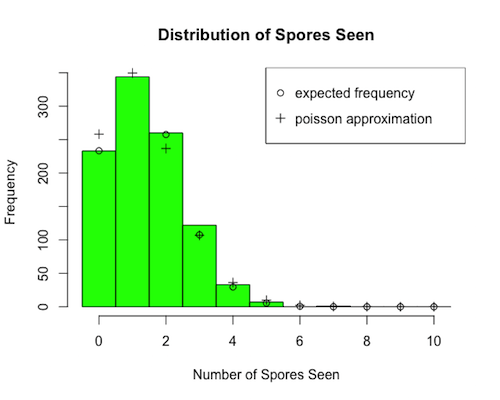## R Project: Testing for AnthraxYou are designing an automated test to see if a 10 mm circular collection plate has accumulated a lethal dose of anthrax (Bacillus Anthracis). A lethal dose of anthrax is 4130 spores, each of which is 0.0001 mm wide and roughly spherical.

Assume you have available to you a computer program that can take pictures of randomly selected regions of the plate using an Olympus Compound microscope set to 1000x magnification (where the field of view is a circle 0.184 mm across), and count the number of anthrax spores present in each picture.

Even working under the assumption that the spores will be uniformly distributed on the plate, given the small field of view you are concerned that a single picture (or perhaps even several pictures) might not reveal the presence of any spores, when they are indeed present on the plate (just not in the places you looked).

1. What is the probability that a single spore on the plate is seen in a randomly selected picture? Write a function in R, named prob.single.spore.seen(p,f,a) that will calculate this value as a function of the plate diameter, $p$, the diameter of the field of view for this microscope, $f$, and the diameter of an anthrax spore, $a$. Assume a spore will be "seen" if any part of it is visible in the field of view.

2. Let X be the number of spores seen in a single randomly selected picture if there are $n$ spores present on the collection plate. What type of distribution does X follow? One of your colleagues insists that for what you will be using it for, the distribution is approximately Poisson in nature. However, given the life-and-death decisions that your work may be called upon to make, you are worried about the potential errors that could be introduced by using an approximating distribution. Consequently, you decide to NOT use a Poisson distribution to model this situation.

Under this restraint, write a function in R, called simulated.data(x,n,p), that simulates the numbers of spores seen in $x$ different randomly selected pictures of a collection plate containing exactly $n$ spores, where the probability of seeing any particular spore is $p$. The result should be a vector of length $x$.

3. You wish to visualize the distribution associated with your simulated data to get a better feel for it. Knowing that the chances of seeing many spores in any one picture will be remote, you wish to plot the left portion of the corresponding frequency histogram where there is a separate bar for each outcome from 0 to 10, but no others.

In the interests of making a nice graphic, you also wish to have a custom title, labels for your x and y axes, and the rectangles of your histogram to be filled with some color other than white or black.

To get a feel for how much variability there is in the frequencies related to any given number of spores seen, you would also like to add points -- one for each rectangle of your histogram -- horizontally centered with respect to their corresponding rectangles and with heights indicating the expected frequencies of seeing the associated number of spores in a given single picture, in accordance with the underlying distribution.

Lastly, as a concession to your colleague, you would like to do the same for the expected frequencies of seeing the associated number of spores in any one picture using the approximating Poisson distribution (to see just how good a job it does in approximating things). So that these Poisson-based frequencies can be distinguished from the true expected frequencies, you decide to plot the former as "plus signs", and the latter as small circles, and to include a legend to indicate which is which.

Write an R function named num.spores.hist(data,n,p,max) that will produce the plot described above, where:

1. data is a vector like that produced by simulated.data(p)
2. n is the number of spores on the collection plate
3. p is the probability of seeing any particular spore in a randomly selected picture, and
4. max is the maximum number of spores you want to account for in your histogram (any simulated numbers of spores seen in your data that are greater than max should be discarded). So for our purposes, we will be interested in running this function when max = 10

Then create the corresponding histogram for 1000 random pictures when there is a lethal dose of anthrax present on the plate (i.e. 4130 spores) by running the following:

p = prob.single.spore.seen(10,0.184,0.0001)
data = simulated.data(1000,4130,p)
num.spores.hist(data,4130,p,10)

4. What would happen if a different magnification level was used -- one that resulted in the field of view being 400 times larger by area, and the number of spores present on the plate was only 10 (i.e., almost 400 times smaller)? Use the function you created previously to produce a plot corresponding to this new situation.

5. Examples of the histograms generated in questions #3 and #4 above are given below. Noticing that the Poisson distribution does a better job at approximating the true distribution in one of these two instances, how might your colleague (correctly) explain why this is the case?

6.7. You wish to know how many different pictures must be examined so that one has at least a 99% chance of seeing at least one spore when a lethal dose (4130 spores) is present. Write an R function, num.pics.needed(n,p) that will determine this value, assuming the answer is not more than 100 pictures (an incredibly conservative estimate). The argument $n$ should be the number of spores on the plate, and $p$ should be the probability of seeing any single particular spore in a randomly selected picture.

p = prob.single.spore.seen(10,0.184,0.0001)